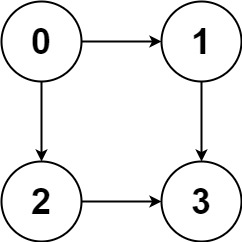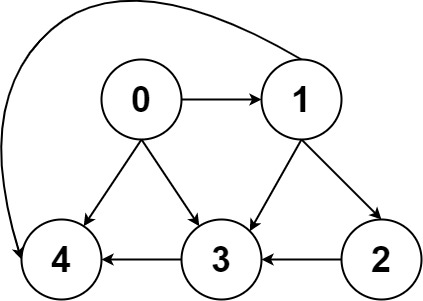## Algorithm

Problem Name: 797. All Paths From Source to Target

Given a directed acyclic graph (DAG) of `n` nodes labeled from `0` to `n - 1`, find all possible paths from node `0` to node `n - 1` and return them in any order.

The graph is given as follows: `graph[i]` is a list of all nodes you can visit from node `i` (i.e., there is a directed edge from node `i` to node `graph[i][j]`).

Example 1:```Input: graph = [[1,2],,,[]]
Output: [[0,1,3],[0,2,3]]
Explanation: There are two paths: 0 -> 1 -> 3 and 0 -> 2 -> 3.
```

Example 2:```Input: graph = [[4,3,1],[3,2,4],,,[]]
Output: [[0,4],[0,3,4],[0,1,3,4],[0,1,2,3,4],[0,1,4]]
```

Constraints:

• `n == graph.length`
• `2 <= n <= 15`
• `0 <= graph[i][j] < n`
• `graph[i][j] != i` (i.e., there will be no self-loops).
• All the elements of `graph[i]` are unique.
• The input graph is guaranteed to be a DAG.

## Code Examples

### #1 Code Example with C++ Programming

```Code - C++ Programming```

``````
class Solution {
public:
vector> allPathsSourceTarget(vector>& graph) {
vector>res;
dfs(graph, res, {}, 0);
return res;
}

void dfs(vector>& graph, vector>& res, vector path, int node){
path.push_back(node);
if(graph[node].size() == 0) res.push_back(path);
for(int neigh: graph[node]) dfs(graph, res, path, neigh);
}
};
``````
Copy The Code &

Input

cmd
graph = [[1,2],,,[]]

Output

cmd
[[0,1,3],[0,2,3]]

### #2 Code Example with Java Programming

```Code - Java Programming```

``````
class Solution {
public List> allPathsSourceTarget(int[][] graph) {
List> list = new ArrayList<>();
int target = graph.length - 1;
List temp = new ArrayList<>();
helper(list, temp, 0, graph, target);
return list;
}

private void helper(List> list, List temp, int curr, int[][] graph, int target) {
if (curr == target) {
}
else {
int[] connections = graph[curr];
for (int connection : connections) {
helper(list, temp, connection, graph, target);
temp.remove(temp.size() - 1);
}
}
}
}
``````
Copy The Code &

Input

cmd
graph = [[1,2],,,[]]

Output

cmd
[[0,1,3],[0,2,3]]

### #3 Code Example with Javascript Programming

```Code - Javascript Programming```

``````
const allPathsSourceTarget = function(graph) {
const res = []
const path = []
bt(graph, res, path, 0)
return res
};

function bt(g, res, path, cur) {
path.push(cur)
if(cur === g.length - 1) res.push(path.slice())
else {
for(let i of g[cur]) bt(g, res, path, i)
}
path.pop()
}
``````
Copy The Code &

Input

cmd
graph = [[4,3,1],[3,2,4],,,[]]

Output

cmd
[[0,4],[0,3,4],[0,1,3,4],[0,1,2,3,4],[0,1,4]]

### #4 Code Example with Python Programming

```Code - Python Programming```

``````
class Solution:
def allPathsSourceTarget(self, graph, i = 0, q = ):
if i == 0:
global res
res = []
if i == len(graph) - 1:
res.append(q)
for index in graph[i]:
self.allPathsSourceTarget(graph, index, q + [index])
return res
``````
Copy The Code &

Input

cmd
graph = [[4,3,1],[3,2,4],,,[]]

Output

cmd
[[0,4],[0,3,4],[0,1,3,4],[0,1,2,3,4],[0,1,4]]

### #5 Code Example with C# Programming

```Code - C# Programming```

``````
using System.Collections.Generic;

namespace LeetCode
{
public class _0797_AllPathsFromSourceToTarget
{
public IList> AllPathsSourceTarget(int[][] graph)
{
var cache = new Dictionary>>();
return AllPathsSourceTarget(graph, 0, cache);
}

private IList> AllPathsSourceTarget(int[][] graph, int node, Dictionary>> cache)
{
if (cache.ContainsKey(node))
return cache[node];

var results = new List>();
foreach (var next in graph[node])
{
if (next == graph.Length - 1)
results.Add(new List() { node, next });

var pathes = AllPathsSourceTarget(graph, next, cache);
if (pathes.Count > 0)
{
foreach (var path in pathes)
{
var newPath = new List();
}
}
}

cache[node] = results;
return results;
}
}
}
``````
Copy The Code &

Input

cmd
graph = [[1,2],,,[]]

Output

cmd
[[0,1,3],[0,2,3]]